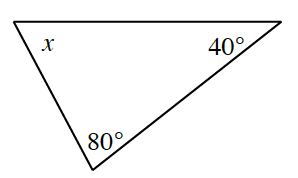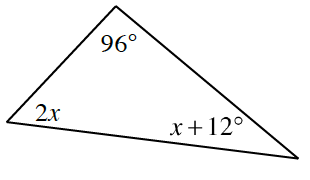### Home > INT2 > Chapter 1 > Lesson 1.3.4 > Problem1-110

1-110.

Use the Triangle Angle Sum Theorem to write an equation and solve for $x$ in each diagram below. Show all work.

1.There are $180$ degrees in every triangle.

1.$2x+96+(x+12)=180$

1. How can you verify that your answer for part (b) is correct?